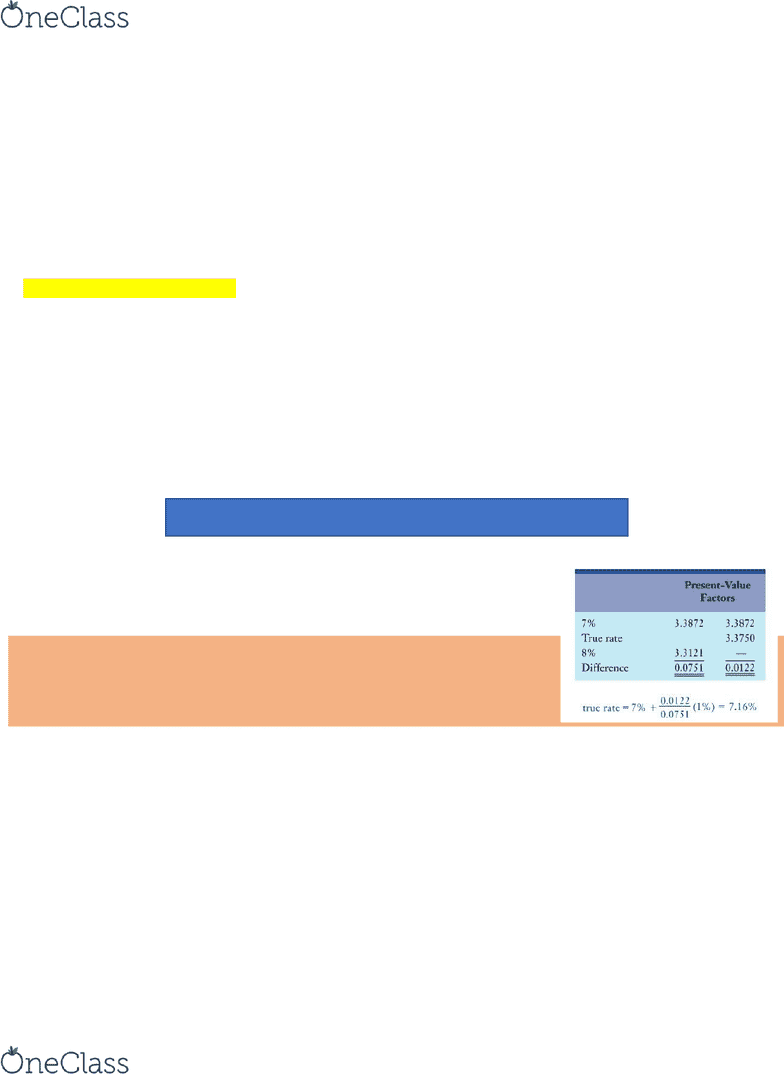Textbook Notes (280,000)
CA (170,000)
COMM (100)
Chapter 10

# COMM-2016EL Chapter Notes - Chapter 10: Cost Of Capital, Net Present Value, Capital Budgeting

Department
Course Code
COMM-2016EL
Professor
Kayla Levesque
Chapter
10

This preview shows pages 1-2. to view the full 7 pages of the document.Chapter Capital Budgeting Decisions
Capital-Budgeting Decisions decisions made in the process of evaluating and choosing among long-term
capital projects
Capital budgeting has 3 phases:
1. Identifying potential investments
2. Selecting the investments to undertake (includes the gathering of data to aid in decision)
3. Follow-up monitoring or “post-audit” of investments
Managers use many different capital-budgeting models in selecting investments, and each model provides
unique information for a decision maker to choose among different investment proposals
Capital Investment Decisions
In planning and controlling, operations managers typically focus on a time-period
Capital Assets used to generate revenues or cost savings that affect more than one year’s financial results
All capital outlays involve risk. The organization must commit funds to the project/program but cannot
be sure what if any returns this investment will yield later ...
Well-managed organizations try to gather and quantify as many known or predictable factors as possible
before making a decision
There are 3 general types of capital-budgeting models: discounted-cash-flow models, payback models, and rate-
of-return models:
Discounted-Cash-Flow Models
Discounted-Cash-Flow (DCF) Model a capital-budgeting model that focuses on cash inflows and outflows,
the time value of money, and identifying criteria for accepting or rejecting capital projects
MAJOR ASPECTS OF DCF
DCF models focus on expected cash inflows and outflows rather than on net income… Companies invest cash
today (cash outflow) to receive cash returns in future periods (cash inflow)
There are 2 main variations of DCF:
a) Net Present Value (NPV)
b) Internal Rate of Return (IRR)
Both variations are based on the theory of compound interest, demonstrated in table 1 & 2 in appendix B
NET PRESENT VALUE (NPV)
Net-Present-Value (NPV) Method an investment evaluation technique that discounts all expected future
cash flows to the present using a minimum desired rate of return
This minimum rate is often called the required rate of return (AKA. hurdle rate, or discount rate)
The minimum desired rate of return depends on the risk of a proposed project the higher the risk, the
higher the minimum desired rate of return
When choosing among several investments, the one with the greatest NPV, notwithstanding qualitative non-
financial considerations, is the most desirable
find more resources at oneclass.com
find more resources at oneclass.com

Only pages 1-2 are available for preview. Some parts have been intentionally blurred.The NPV method is applied as follows:
1. Prepare a list of all expected cash inflows and outflows, preferably in chronological order starting with the
initial investment at the time of the acquisition of the capital asset at time zero
2. Find the present value of each expected cash inflow and outflow
3. Total up the individual present values calculated in step 2. If the total is negative, reject the project; if it is
positive, accept the project
o A positive NPV means that accepting the project will increase the value of the firm because the
PV of the project’s cash inflows exceeds the PV of its cash outflows
o If NPV were 0, we would be indifferent
INTERNAL RATE OF RETURN (IRR)
Internal Rate of Return (IRR) the discount rate that makes the net present value of the project equal to 0
If the IRR is greater than the minimum desired rate of return, a project is to be accepted
If the IRR is lower than the minimum desired rate of return, a project is to be rejected
The 3 steps in calculating IRR are:
1. Prepare a diagram of the expected cash inflows and outflows just like in calculating NPV
2. Find an interest rate that equates the present value of the cash inflows to the present value of the cash
outflows; that is, produces an NPV of 0
3. Compare the IRR with the minimum desired rate of return
If one outflow is followed by a series of equal inflows, you can use the following equation:
Initial Investment = Annual Cash Flow x Annuity PV Factor (F)
Not all IRR calculations work out exactly… To obtain a more accurate rate,
interpolation is needed
For example, let’s say F = 3.3750. In the table, the column closest to 3.3750 is
7%, which may be close enough for most purposes… The factor 3.3750 is
between the 7% factor and the 8% factor
ASSUMPTIONS OF DCF MODELS
Two major assumptions underlie DCF models…
We assume a world of uncertainty. Therefore, we act as if the predicted cash inflows and outflows are
certain to occur at the times specified
We assume perfect capital markets. That is, if we have extra cash at any time, we can borrow or lend
money at the same interest rate (this rate is our min. desired rate of return for NPV and IRR for IRR)
If these assumptions are met, no model could possibly be better than a DCF model. Unfortunately, our world
has neither certainty nor perfect capital markets. Therefore, NPV and IRR, although good, are not perfect
models. Nevertheless, the DCF model is usually preferred to other models
find more resources at oneclass.com
find more resources at oneclass.com